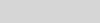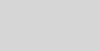# Some UnicodeMath EnhancementsMurray Sargent

In the years since UnicodeMath 3.1 was published, some improvements have been made. The converter that converts UnicodeMath to OfficeMath also converts LaTeX and Nemeth math braille to OfficeMath. The converter needs ways to provide OfficeMath math-object arguments even when these arguments are not marked as such in the math format. The resulting infrastructure is available for converting all three formats to OfficeMath.

### n-aryands

With all three formats, the n-aryand, e.g., integrand or summand, may not be identified by surrounding delimiters. But OfficeMath and MathType have n-aryand arguments as described in the post Integrands, Summands, and Math Function Arguments. UnicodeMath has the binary operator U+2592 (▒) to treat the expression that follows the ▒ as the n-aryand (see Section 3.4 of UnicodeMath 3.1). In generalizing the conversion code for LaTeX and braille, it became clear that a space alone is adequate for starting n-aryands and we don’t need the ▒, which doesn’t look like mathematics. So, the converter now makes the first expression that follows the n-ary operator and limits into the n-aryand. For example, the integralcan be given by the UnicodeMath 1/2π ∫_0^2π ⅆθ/(a+b sin θ)=1/√(a^2-b^2) since the first expression that follows the ∫_0^2π is the fraction ⅆθ/(a+b sin θ). This works for many integrands. More complicated integrands are usually enclosed in brackets, braces, or parentheses.

### Matrix improvements

A “bare” matrix, that is, one with no enclosing brackets can be entered by typing the TeX control word \matrix. In addition, there are five matrix constructs with enclosing brackets that can be entered as summarized in the following table in which … stands for the matrix contents.

 LaTeX Char Code Form \matrix ■ U+25A0 … \bmatrix ⓢ U+24E2 […] \pmatrix ⒨ U+24A8 (…) \vmatrix ⒱ U+24B1 |…| \Bmatrix Ⓢ U+24C8 {…} \Vmatrix ⒩ U+24A9 ‖…‖

The UnicodeMath syntax for a parenthesized 2×2 matrix is \pmatrix(a&b@c&d), which builds up asSometimes you just want to enter a sample matrix quickly. If any of the six matrix control words are followed by a digit d, they insert a d × d identity matrix. For example, typing \pmatrix 3 entersThis is easier to type than \pmatrix(1&0&0@0&1&0@0&0&1), which displays the same identity matrix. Some of the matrix control words are missing in the default math autocorrect file. You can add them as described in the last section of this post.

## sin 𝑥²

This trigonometric expression is ambiguous: is it sin(𝑥²) or (sin 𝑥)²? Without the parentheses, the UnicodeMath for the former is “sin x^2” and for the latter is “sin x ^2”. In the latter, the space following the x builds up the sin x into a math function object and then the ^2 squares the object. But the results are very different formulas. The converter avoids the ambiguity by building up “sin x ^2” to be the same math function object as “sin^2 x”, that is, sin² 𝑥.

### Some LaTex in UnicodeMath mode

You can enter the common LaTeX expressions \frac{a}{b} and \binom{n}{m} in UnicodeMath input mode provided you have added math autocorrect entries to convert \frac to ⍁ (U+2341) and \binom to ⒝ (U+249D). To add math autocorrect entries, click on the lower-right box in the Equations/Conversions ribbon option to display the dialog boxThen click on the Math AutoCorrect… button to see and add math autocorrect entries. For example, to add \frac with U+2341, type as in the dialog boxAnd then enter Alt+x to convert the 2341 to ⍁. Probably when you type LaTeX in UnicodeMath input mode, a dialog ought to appear asking you if you’d like to switch to LaTeX input mode.

•D. Jakov 0

A little summary of Ideas for possible future improvements, and some bug reports

First of all, thank you for your amazing work, your efforts in creating and developing Office Math and maintaining this Blog. Here are a few thoughts and ideas I gathered during the last two years of extensively using Unicode Math and the LaTeX- Syntax in MS Word:

1) Current bug in PowerPoint:
“Math font with exponent can not be displayed correctly in MS PowerPoint”, posted as an Issue on GitHub, see here:
https://github.com/alerque/libertinus/issues/465
This might be improved.

2) Ability to change positions of the limits of a sum inside a fraction.
In LaTeX this can be achieved by the command \limits, in Office Math it is not possible.
Example (LaTeX- Syntax):
a=\frac{\sum_{i=1}^{n}{\ \left(x_i-\overline{x}\right)\left(y_i-\overline{y}\right)}}{\sum_{i=1}^{n}\left(x_i-\overline{x}\right)^2}
Unfortunately this looks very dense with the limits in front of the sum, it would look much better if they were above and below the summation symbol, even if in a fraction.

3) Enabling the usage of stylistic sets in Math Zones when working with Opentype Math Fonts (other than Cambria Math).
Currently I use the Opentype Math Font “Libertinus Math”, which offers two variants of the \int – symbol; the default integration symbol is upright, but there is also a slanted variant. In LaTeX this can be easily adjusted, but the attempt to use the stylistic set +ss08 in MS Word inside Math Zones fails. However, in text mode it works. It would be grate if Word allows this kind of flexibility in the future. ( Other fonts like STIX Two Math have two different version of the integral sign as well, which a Word user might want to be able to switch)

4) Bug, or at least a non- ideal behaviour: When one creates a PDF – file from a word document, that contains (mathematical) text created with fonts installed as otf, these contents are embedded as bitmaps instead of vector graphics inside the PDF. A contributor in a Microsoft community forum has summarized the problem and the wish of its improvement as follows “The issue lies in how Word handles open type fonts.  They come in two flavors, opentype with true type outlines, and opentype with postscript outlines.  Word (usually) correctly embeds the true type flavor but can’t do the postscript outlines type so renders them to bitmaps (thus making them look a bit rubbish, Boooo!) A solution if the font licence allows it is to use an online converter to convert your .otf font to .ttf, this will convert your the curves from cubic (postscript type) curves to quadratic (true type) curves and then install that. My experience is Word wil happily embed them once that is done. Of course the ideal would be if Word behaved and embeded both flavours of font nicely ;)”

I know the issues and topics listed above, might not be a priority of you and the development team, but to at least consider improving some of them would be amazing. Keep up the good work 🙂

•anonymous 0

this comment has been deleted.